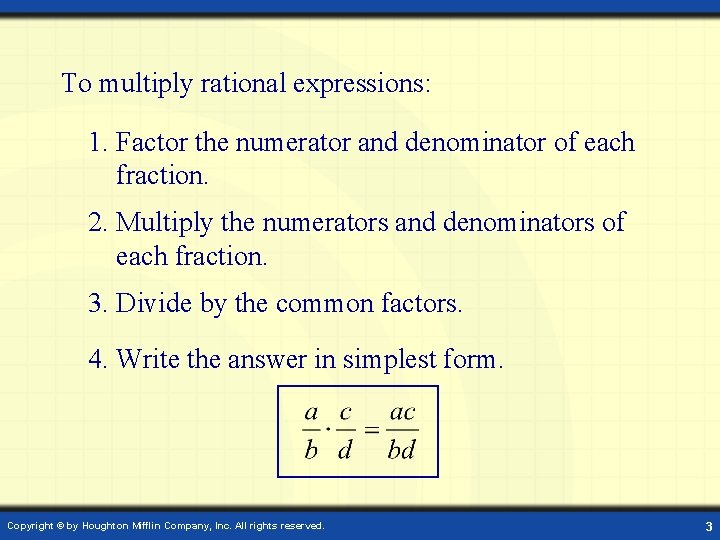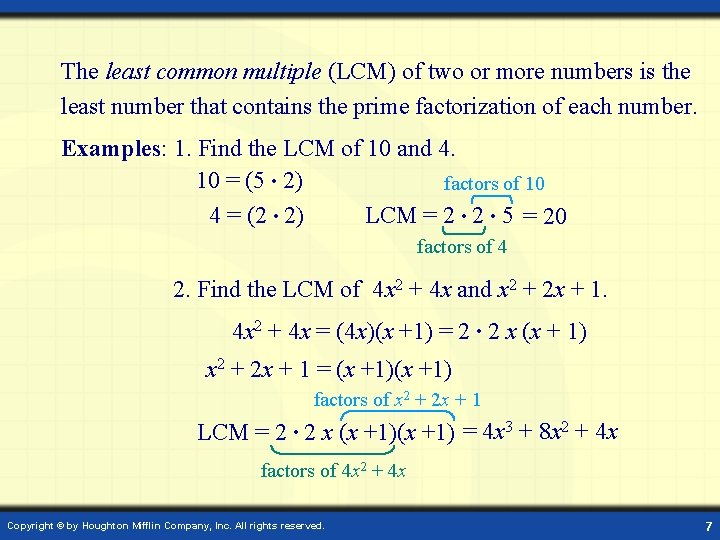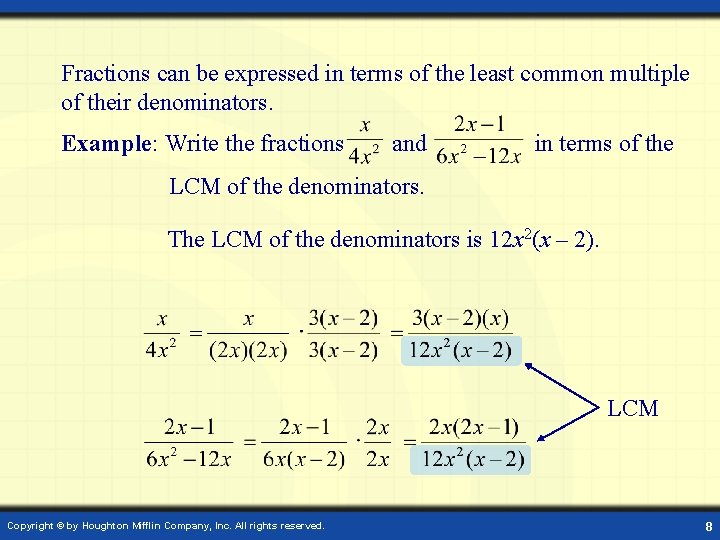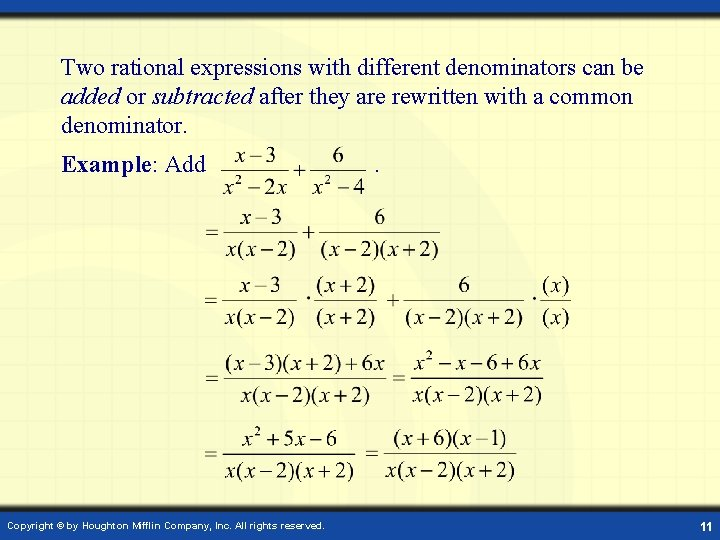# Digital Lesson Operations on Rational Expressions Rational expressions

• Slides: 12Digital Lesson Operations on Rational ExpressionsRational expressions are fractions in which the numerator and denominator are polynomials and the denominator does not equal zero. Example: Simplify . , x– 3 0 , x 3 Copyright © by Houghton Mifflin Company, Inc. All rights reserved. 2To multiply rational expressions: 1. Factor the numerator and denominator of each fraction. 2. Multiply the numerators and denominators of each fraction. 3. Divide by the common factors. 4. Write the answer in simplest form. Copyright © by Houghton Mifflin Company, Inc. All rights reserved. 3Example: Multiply . Factor the numerator and denominator of each fraction. Multiply. Divide by the common factors. Write the answer in simplest form. Copyright © by Houghton Mifflin Company, Inc. All rights reserved. 4To divide rational expressions: 1. Multiply the dividend by the reciprocal of the divisor. The reciprocal of is . 2. Multiply the numerators. Then multiply the denominators. 3. Divide by the common factors. 4. Write the answer in simplest form. Copyright © by Houghton Mifflin Company, Inc. All rights reserved. 5Example: Divide . Multiply by the reciprocal of the divisor. Factor and multiply. Divide by the common factors. Simplest form Copyright © by Houghton Mifflin Company, Inc. All rights reserved. 6The least common multiple (LCM) of two or more numbers is the least number that contains the prime factorization of each number. Examples: 1. Find the LCM of 10 and 4. 10 = (5 • 2) factors of 10 4 = (2 • 2) LCM = 2 • 5 = 20 factors of 4 2. Find the LCM of 4 x 2 + 4 x and x 2 + 2 x + 1. 4 x 2 + 4 x = (4 x)(x +1) = 2 • 2 x (x + 1) x 2 + 2 x + 1 = (x +1) factors of x 2 + 2 x + 1 LCM = 2 • 2 x (x +1) = 4 x 3 + 8 x 2 + 4 x factors of 4 x 2 + 4 x Copyright © by Houghton Mifflin Company, Inc. All rights reserved. 7Fractions can be expressed in terms of the least common multiple of their denominators. Example: Write the fractions and in terms of the LCM of the denominators. The LCM of the denominators is 12 x 2(x – 2). LCM Copyright © by Houghton Mifflin Company, Inc. All rights reserved. 8To add rational expressions: 1. If necessary, rewrite the fractions with a common denominator. 2. Add the numerators of each fraction. To subtract rational expressions: 1. If necessary, rewrite the fractions with a common denominator. 2. Subtract the numerators of each fraction. Copyright © by Houghton Mifflin Company, Inc. All rights reserved. 9